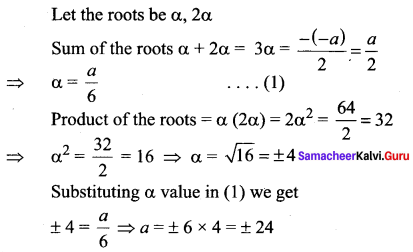Tamilnadu Samacheer Kalvi 11th Maths Solutions Chapter 2 Basic Algebra Ex 2.4

11th Maths Exercise 2.4 Solutions Question 1.
Construct a quadratic equation with roots 7 and -3.
Solution:
The given roots are 7 and -3
Let α = 7 and β = -3
α + β = 7 – 3 = 4
αβ = (7)(-3) = -21
The quadratic equation with roots α and β is x2 – (α + β) x + αβ = 0
So the required quadratic equation is
x2 – (4) x + (-21) = 0
(i.e.,) x2 – 4x – 21 = 0

11th Maths Exercise 2.4 Question 2.
A quadratic polynomial has one of its zeros 1 + $$\sqrt{5}$$ and it satisfies p(1) = 2. Find the quadratic polynomial.
Solution:
Given α = 1 + $$\sqrt{5}$$ So, β = 1 – $$\sqrt{5}$$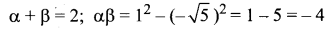p(x) = x2 – (α + β)x + αβ
p(x) = k (x2 – 2x – 4)
p( 1) = k(1 – 2 – 4) = -5 k
Given p (1) = 2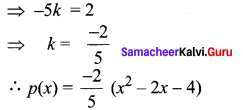Exercise 2.4 Class 11 Maths Question 3.
If α and β are the roots of the quadratic equation x2 + $$\sqrt{2}$$x + 3 = 0, form a quadratic polynomial with zeroes 1/α, 1/β.
Solution:
α and β are the roots of the equation x2 + $$\sqrt{2}$$x + 3 = 0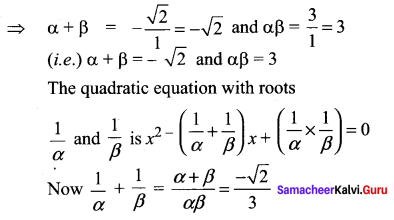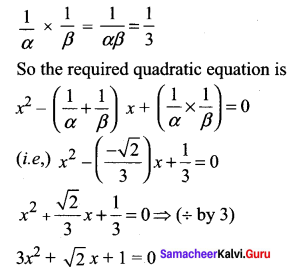11th Maths Exercise 2.4 Answers Question 4.
If one root of k(x – 1)2 = 5x – 7 is double the other root, show that k = 2 or – 25.
Solution:
k(x – 1)2 = 5x – 7
(i.e.,) k(x2 – 2x + 1) – 5x + 7 = 0
x2 (k) + x(-2k – 5) + k + 1 = 0
kx2 – x(2k + 5) + (k + 7) = 0
Here it is given that one root is double the other.
So let the roots to α and 2α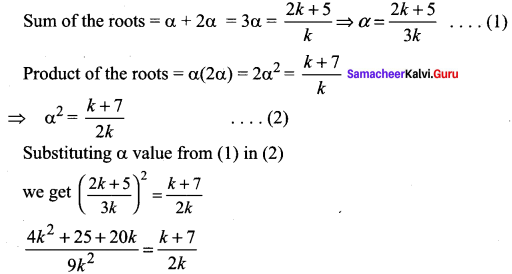2(4k2 + 25 + 20k) = 9k (k + 7)
2(4k2 + 25 + 20k) = 9k2 + 63k
8k2 + 50 + 40k – 9k2 – 63k = 0
-k2 – 23k + 50 = 0
k2 + 23k – 5o = 0
(k + 25)(k – 2) = 0
k = -25 or 2

Exercise 2.4 Class 11 Question 5.
If the difference of the roots of the equation 2x2 – (a + 1)x + a – 1 = 0 is equal to their product then prove that a = 2.
Solution: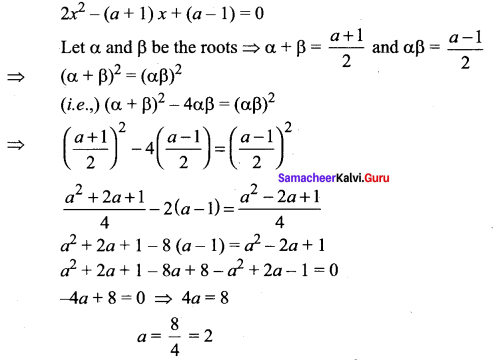Samacheer Kalvi 11th Maths Solution Question 6.
Find the condition that one of the roots of ax2 + bx + c may be
(i) negative of the other
(ii) thrice the other
(iii) reciprocal of the other.
Solution:
(i) Let the roots be α and -β
Sum of the roots = – b/a = 0 ⇒ b = 0

(ii) Let the roots be α, 3α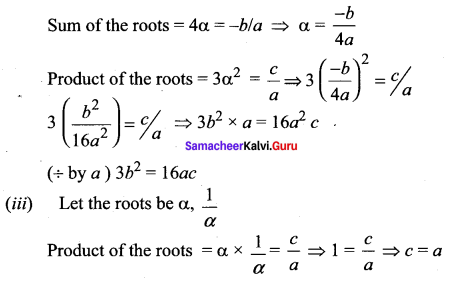Samacheer Kalvi 11 Maths Solutions Question 7.
If the equations x2 – ax + b = 0 and x2 – ex + f = 0 have one root in common and if the second equation has equal roots that ae = 2(b + f).
Solution: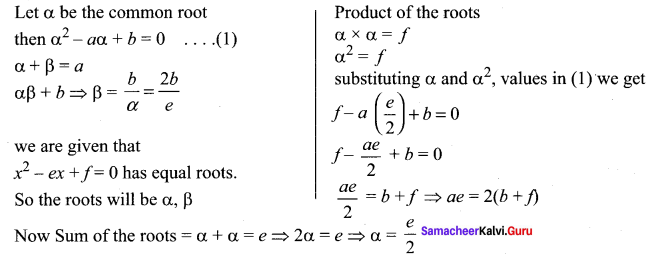Samacheer Kalvi Guru 11th Maths Question 8.
Discuss the nature of roots of
(i) -x2 + 3x + 1 = 0
(ii) 4x2 – x – 2 = 0
(iii) 9x2 + 5x = 0
Solution:
(i) -x2 + 3x + 1 = 0
⇒ comparing with ax2 + bx + c = 0
∆ = b2 – 4ac = (3)2 – 4(1)(-1) = 9 + 4 = 13 > 0
⇒ The roots are real and distinct

(ii) 4x2 – x – 2 = 0
a = 4, b = -1, c = -2
∆ = b2 – 4ac = (-1)2 – 4(4)(-2) = 1 + 32 = 33 >0
⇒ The roots are real and distinct

(iii) 9x2 + 5x = 0
a = 9, b = 5, c = 0
∆ = b2 – 4ac = 52 – 4(9)(0) = 25 > 0
⇒ The roots are real and distinct

Samacheer Kalvi 11th Maths Solutions Question 9.
Without sketching the graphs find whether the graphs of the following functions will intersect the x- axis and if so in how many points.
(i) y = x2 + x + 2
(ii) y = x2 – 3x – 1
(iii) y = x2 + 6x + 9
Solution: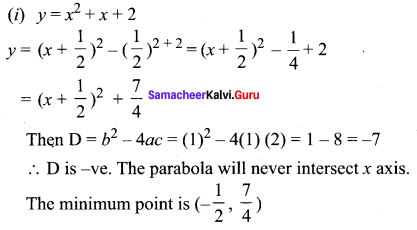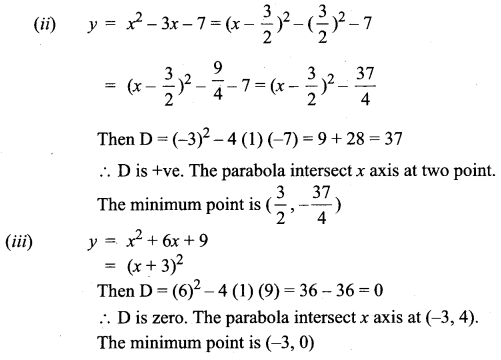Samacheer Kalvi Class 11 Maths Solutions Question 10.
Write f(x) = x2 + 5x + 4 in completed square form.
Solution: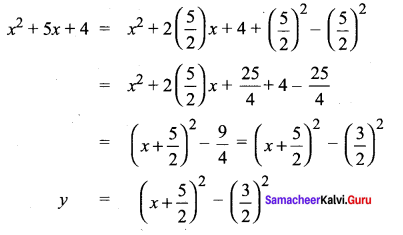Samacheer Kalvi 11th Maths Solutions Chapter 2 Basic Algebra Ex 2.4 Additional Questions

11th Maths Solutions Samacheer Kalvi Question 1.
Find the values of k so that the equation x2 = 2x (1 + 3k) + 7(3 + 2k) = 0 has real and equal roots.
Solution:
The equation is x2 – x(2) (1 + 3k) + 7 (3 + 2&) = 0
The roots are real and equal
⇒ ∆ = 0 (i.e.,) b2 – 4ac = 0
Here a = 1, b = -2 (1 + 3k), c = 7(3 + 2k)
So b2 – 4ac = 0 ⇒ [-2 (1 + 3k)]2 – 4(1) (7) (3 + 2k) = 0
(i.e.,) 4 (1 + 3k)2 – 28 (3 + 2k) = 0
(÷ by 4) (1 + 3k)2 – 7(3 + 2k) = 0
1 + 9k2 + 6k – 21 – 14k = 0
9k2 – 8k – 20 = 0
(k – 2)(9k + 10) = 0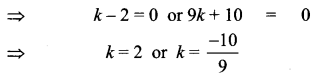To solve the quadratic inequalities ax2 + bx + c < 0 (or) ax2 + bx + c > 0

11th Maths Samacheer Kalvi Solutions Question 2.
If the sum and product of the roots of the quadratic equation ax2 – 5x + c = 0 are both equal to 10 then find the values of a and c.
Solution:
The given equation is ax2 – 5x + c = 0
Let the roots be α and β Given α + β = 10 and αβ = 10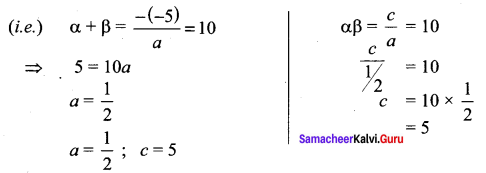Samacheer Kalvi 11th Maths Question 3.
If α and β are the roots of the equation 3x2 – 4x + 1 = 0, form the equation whose roots are $$\frac{\alpha^{2}}{\beta}$$ and $$\frac{\beta^{2}}{\alpha}$$
Solution: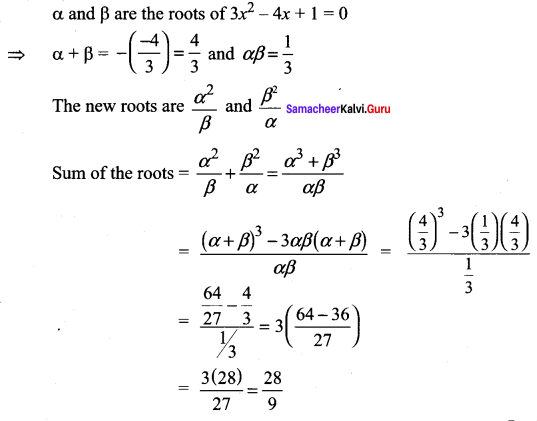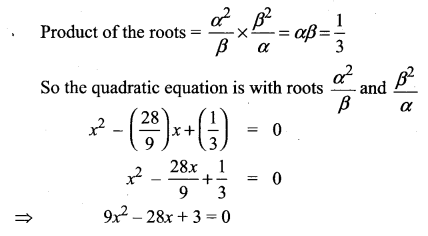11th Maths Chapter 2 Solution Samacheer Kalvi Question 4.
If one root of the equation 3x2 + kx – 81 = 0 is the square of the other then find k.
Solution: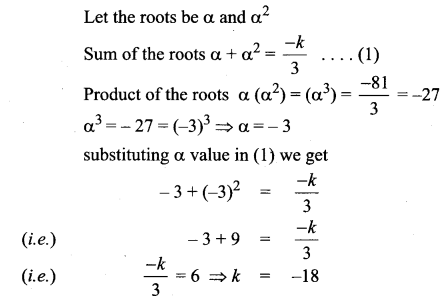Samacheer Kalvi Guru 11th Maths Solution Question 5.
If one root of the equation 2x2 – ax + 64 = 0 is twice that of the other then find the value of a
Solution: